This menu is active only when a table is selected. These commands allow plotting data from the selected table. As a group, all of these commands create a new graph window which contains a single, empty layer. The newly created plot is drawn on this empty layer.

## Plot Wizard (Ctrl-Alt-W)

Opens the Plot Wizard dialog.

## Line ->

Selecting Line -> opens up a sub-menu of additional commands for plotting specialized graphs consisting of lines.

### Line

Plots the selected data columns using "Line" style. The command can also be activated by clicking on theicon of the Table toolbar. Once the plot is created, the drawing style of the data series can be customized with the Custom curves dialog.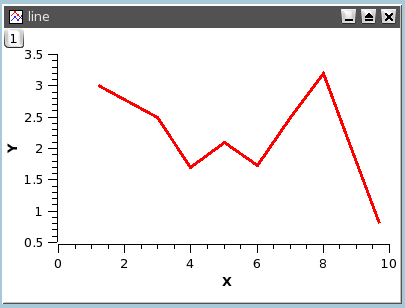### Line Central

Plots a graph of the selected data columns using "Line" style and the X and Y axes located in the middle of the layer. The command can also be activated by clicking on theicon of the Table toolbar. Once the plot is created, the drawing style of the data series can be customized with the Custom curves dialog. The axes can also be customized by opening the corresponding dialog via a double-click on the axis ticks or labels.

### Vertical Steps

Plots the selected data columns using "Vertical Steps" style. Once the plot is created, the drawing style of the data series can be customized with the Custom curves dialog.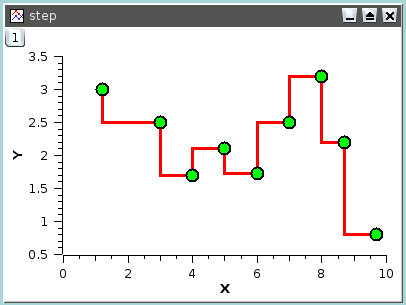### Horizontal Steps

Plots the selected data columns using "Horizontal Steps" style. Once the plot is created, the drawing style of the data series can be customized with the Custom curves dialog.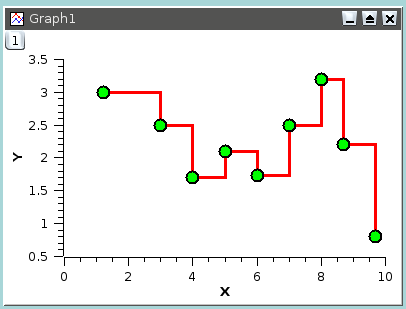## Symbol ->

Selecting Symbol -> opens up a sub-menu of additional commands for plotting specialized graphs using "Scatter" style for the data.

### Scatter

Similar to the Line command except that the plot is drawn using "Scatter" style. The command can also be activated by clicking on theicon of the Table toolbar. Once the plot is created, the drawing style of the data series can be customized with the Plot details dialog.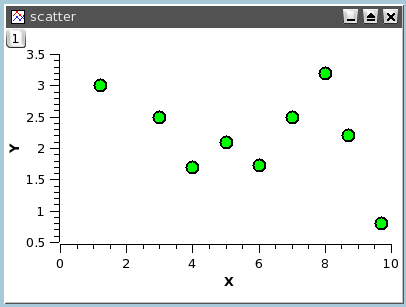### Scatter Central

Plots a graph of the selected data columns using "Scatter" style and the X and Y axes located in the middle of the layer. The command can also be activated by clicking on the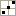icon of the Table toolbar. Once the plot is created, the drawing style of the data series can be customized with the Plot details dialog. The axes can also be customized by opening the corresponding dialog via a double-click on the axis ticks or labels.### Vertical Drop Lines

Plots the selected data columns using "Vertical drop lines" style. The command can also be activated by clicking on the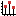icon of the Table toolbar. Once the plot is created, the drawing style of the data series can be customized with the Plot details dialog.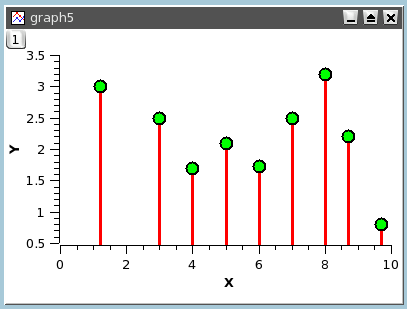### Bubble

Plots the selected data columns using indexed sizes for the plot symbols. You need to select at least two Y columns for this plot operation. The first Y column in the selection is used for the positions of the plot symbols and the next one determines their sizes. The command can also be activated by clicking on theicon of the Table toolbar. Once the plot is created, the drawing style of the data series can be customized with the Plot details dialog.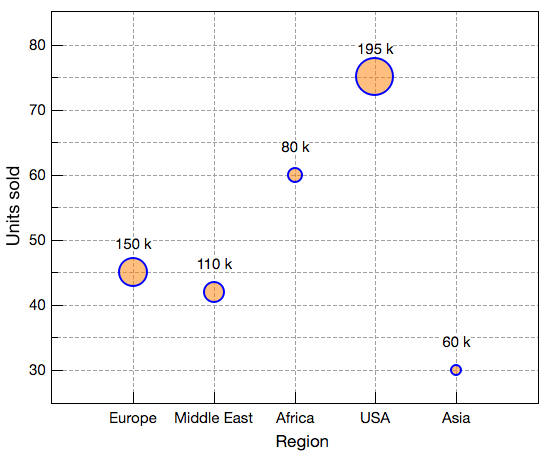### Color Mapped

Plots the selected data columns using a default color map for the plot symbols. You need to select at least two Y columns for this plot operation. The first Y column in the selection is used for the positions of the plot symbols and the next one determines their color. QtiPlot finds the minimum and maximum values in the second Y column, creates eight evenly sized ranges of values between the minimum and maximum values, and then associates a color with each range of values. Each data point color is determined by finding the color associated with the second Y column value in the color map.

The command can also be activated by clicking on theicon of the Table toolbar. Once the plot is created, the drawing style of the data series can be customized with the Plot details dialog.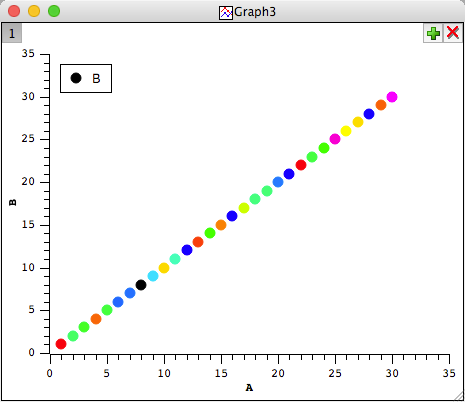### Bubble + Color Mapped

Plots the selected data columns using indexed sizes and a default color map for the plot symbols. You need to select at least two Y columns for this plot operation. The first Y column in the selection is used for the positions of the plot symbols and the next one determines their sizes. If the selection contains three Y columns the third column controls the symbol color: QtiPlot finds the minimum and maximum values in this Y column, creates eight evenly sized ranges of values between the minimum and maximum values, and then associates a color with each range of values. Each data point color is determined by finding the color associated with the second Y column value in the color map. If the column selection contains only two Y columns the second column controls both the size and color of the symbols.

The command can also be activated by clicking on the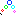icon of the Table toolbar. Once the plot is created, the drawing style of the data series can be customized with the Plot details dialog.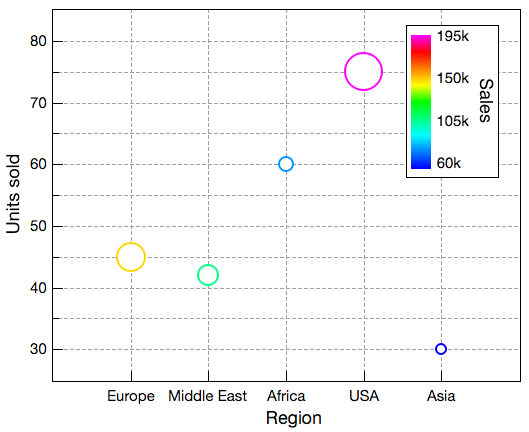## Line + Symbol ->

### Line+Symbol

Identical to the Line command except that the plot is drawn using "Line + Symbol" style. This command can also be activated by clicking on theicon of the Table toolbar. Once the plot is created, the drawing style of the data series can be customized with the Custom curves dialog.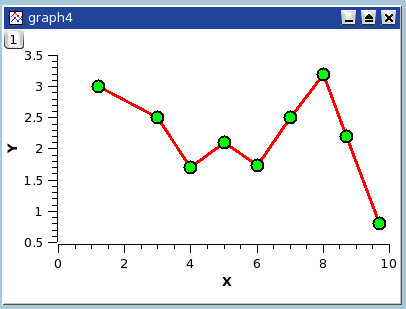### Line + Symbol Central

Plots a graph of the selected data columns using "Line + Symbol" style and the X and Y axes located in the middle of the layer. The command can also be activated by clicking on the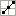icon of the Table toolbar. Once the plot is created, the drawing style of the data series can be customized with the Custom curves dialog. The axes can also be customized by opening the corresponding dialog via a double-click on the axis ticks or labels.

### Spline

Plots the selected data columns using "Spline" style. Once the plot is created, the drawing style of the data series can be customized with the Custom curves dialog.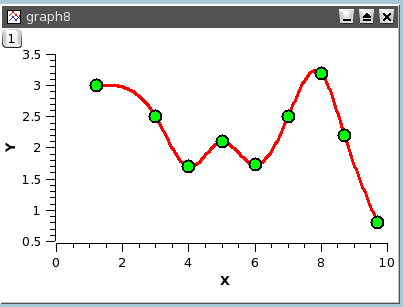## Column/Bar/Pie ->

Selecting Column/Bar/Pie opens up a sub-menu of commands for plotting specialized bar and column graphs.

### Columns

Plots the selected data columns using "Columns" style, that is vertical bars. By default the baseline for the columns is the Y = 0 axis. It is possible to define a different baseline value via the plot details dialog.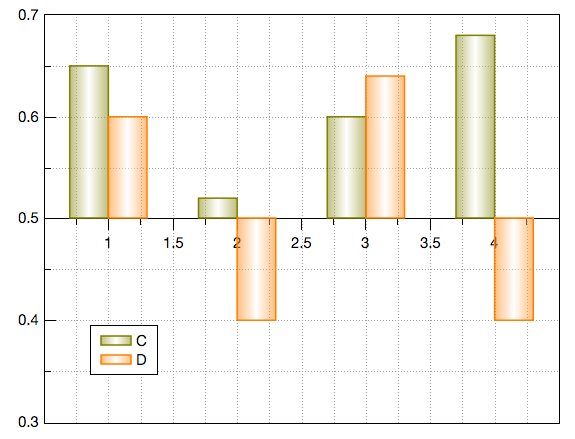### Rows

Plots the selected data columns using "Rows" style. By default the baseline for the rows is the X = 0 axis. It is possible to define a different baseline value via the plot details dialog.### Stack Column

This command can be activated by clicking on theicon of the Table toolbar. The Stack Column command plots a stack-column for each selected row in the currently active table. It is essentially the same as the Stack Bar plot, but drawn as vertical stack-columns rather than horizontal stack-bars.

### Stack Bar

This command can be activated by clicking on theicon of the Table toolbar. The Stack Bar command plots a stack-bar for each selected row in the currently active table. They may be used to conveniently display cumulative data that changes over time, such as budgetary information. The stack-bars are drawn one above the other in a position determined by the X value of the corresponding row. Each stack-bar is composed of a set of joined segments. The widths of the segments are set by the Y values in the row and their relative positions correspond to the column order in the table. Each segment is plotted in a color corresponding to the column number of the Y value determining the segment's width. Colors, segment outlining, vertical separation of the stack-bars, and the axis values can all be customized. An example of a stack-bar plot is shown below, followed by the table that was used to generate the plot.A stack-bar plot showing a hypothetical budgetary breakdown for a 5 year period. The data in the table shown below was used to generate this plot.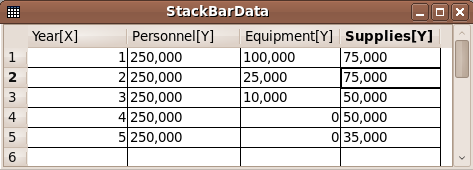### 100% Stack Column

This command can be activated by clicking on theicon of the Table toolbar. The 100% Stack Column command plots a stack-column for each selected row in the currently active table. It is essentially the same as the 100% Stack Bar plot, but drawn as vertical stack-columns rather than horizontal stack-bars.

### 100% Stack Bar

This command can be activated by clicking on the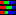icon of the Table toolbar. The 100% Stack Bar command plots a stack-bar for each selected row in the currently active table, comparing the percentage that each value contributes to a total across categories. You can use a 100% stacked bar plot when you have several data sets and you want to emphasize the contributions to the whole, especially if the total is the same for each category.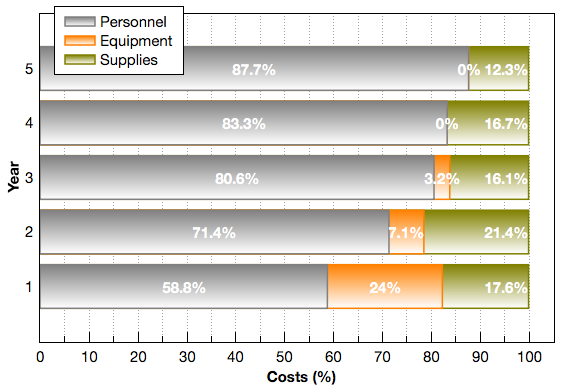### Floating Column

This command can be activated by clicking on the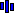icon of the Table toolbar. The floating column graph displays Y values as beginning and ending column levels for each X value. You must select at least two Y columns of values (or a range from at least two columns). If there is an X column to the left of the Y columns, the X column values are used; otherwise, the worksheet's default X values are used.

### Floating Bar

This command can be activated by clicking on theicon of the Table toolbar. The floating bar graph displays Y values as beginning and ending bar levels for each X value. The X values are plotted along the vertical axis. You must select at least two Y columns of values (or a range from at least two columns). If there is an X column to the left of the Y columns, the X column values are used; otherwise, the worksheet's default X values are used.

### 3D Color Pie Chart

This command can be activated by clicking on the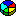icon of the Table toolbar. Creates a pseudo 3D colored pie chart using the selected column in the active table window (only one column allowed).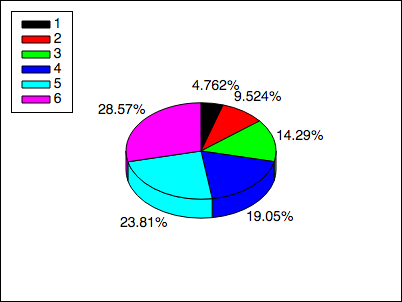### 2D Color Pie Chart

This command can be activated by clicking on theicon of the Table toolbar. Creates a 2D colored pie chart using the selected column in the active table window (only one column allowed).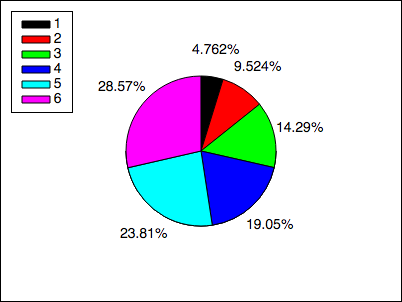### Doughnut

This command can be activated by clicking on theicon of the Table toolbar. Creates a 2D colored doughnut chart using the selected columns in the active table window.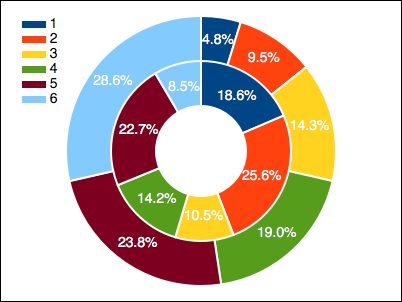## Multi-Curve ->

Selecting Multi-Curve -> opens up a sub-menu of additional commands for plotting multiple data sets.

### Double-Y

Creates a double Y axis graph. Requires a selection of at least two Y columns (or a range from at least two columns). The last Y column in the selection range is attached to the right axis of the plot layer.### 3Y: Y-YY

Creates a three Y axes graph. Requires a selection of at least three Y columns (or a range from at least three columns). In order to display a third Y axis QtiPlot uses two plot layers with overlapping drawing canvases. The right axis of the second plot layer is displayed using a horizontal offset with respect to the drawing canvas. The last data curve created from the selected range is attached to this axis.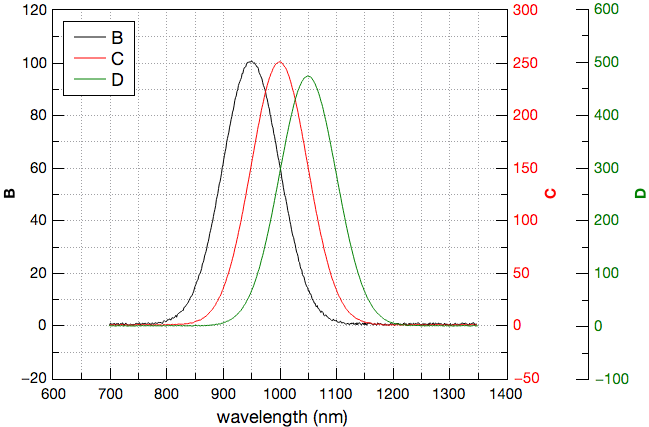### 4Y: YY-YY

Creates a four Y axes graph. Requires a selection of at least four Y columns (or a range from at least four columns). Two overlapping layers are created for this type of plot. Both Y axes of the second plot layer are enabled.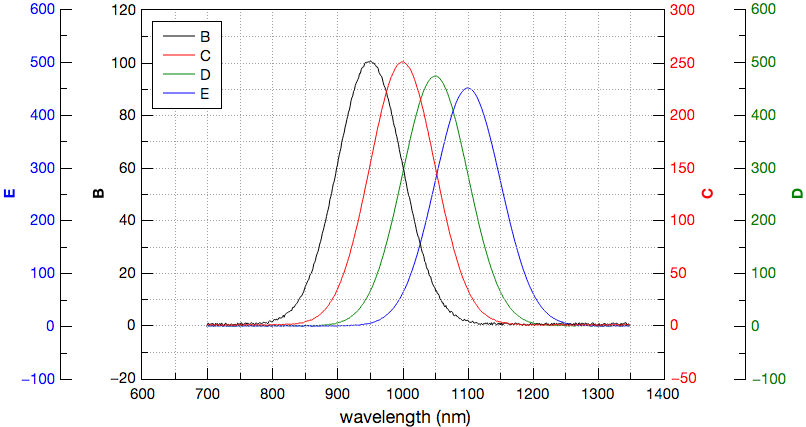### Stack Lines by Y Offsets

Creates a new graph window containing a Stacked Lines plot generated from data in the selected columns. Each column is drawn as a separate curve which has a vertical offset from the previous one. This offset setting that can be modified in the Stack tab of the Plot Details dialog. By default, Stacked Lines by Y Offsets graphs employ the "Auto" data plot offset type, meaning that a gap of 8% is automatically calculated between each data plot. The distances between each curve can be adjusted using this offset feature to eliminate the need to change the original data. Using this feature prevents the curves from overlapping and ensures that each curve can be viewed clearly.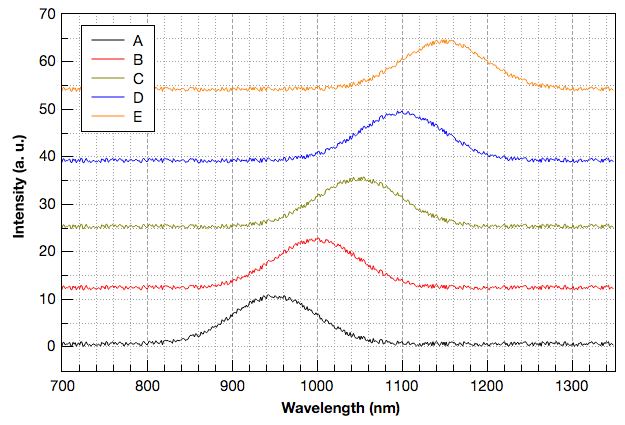### Waterfall

Creates a new graph window containing a waterfall plot generated from data in the selected columns. Each column is drawn as a separate curve which is offset from the previous one in both X and Y, creating the so-called waterfall effect. The figure shown below is a simple example of a waterfall plot where each column is simply an attenuated version of the previous column.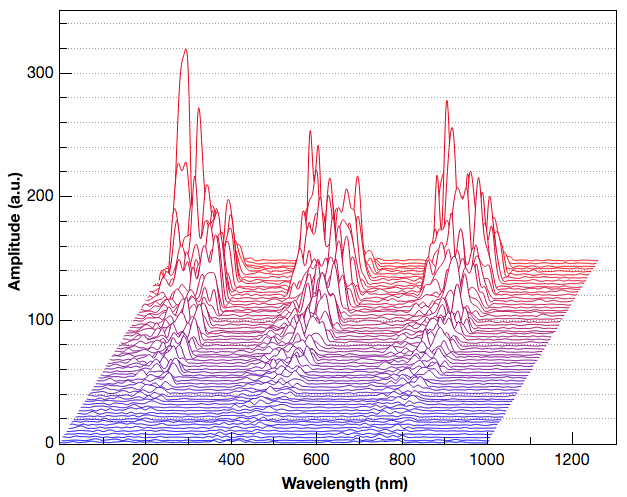### Colormapped Lines

Requires a selection of at least two Y columns (or a data range from at least two columns). Creates a new graph window displaying each data set as a line curve. Existing column comments are treated as Z values if they are valid floating point values. If there are no valid column comments, the column indices define the Z values. The colors of the curves are mapped to these Z values and a color scale legend is added at the right side of the plot layer.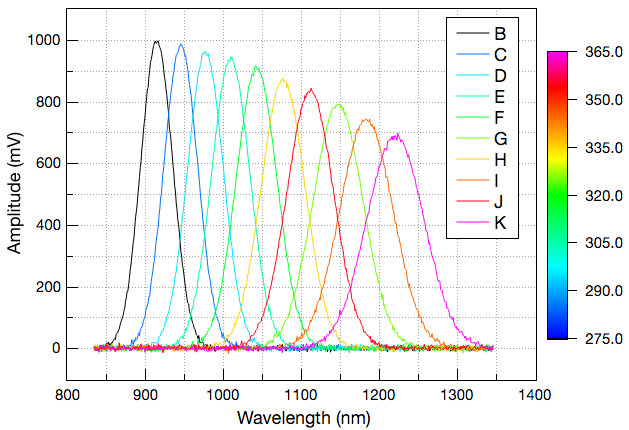## Area ->

Selecting Area -> opens up a sub-menu of additional commands for generating area graphs.

### Area

An Area graph is a line graph with the areas below the lines filled with colors. This command can be activated by clicking on theicon of the Table toolbar. You must select at least one Y column of values (or a range from at least one column). If the Y column(s) has an associated X column, the X column supplies the X values; otherwise, the worksheet's default X values are used.

By default the baseline for the area is the Y = 0 axis. It is possible to define a different baseline value via the plot details dialog.### Stack Area

This command can be activated by clicking on theicon of the Table toolbar. In order to create this type of graph you must select at least two Y columns of values (or a range from at least two columns). Each successive column of values is displayed as an area graph that is stacked on the previous area graph. The upper bounds of the first area graph is treated as the baseline of the next area graph.

### 100% Stack Area

This command can be activated by clicking on theicon of the Table toolbar. In order to create this type of graph you must select at least two Y columns of values (or a range from at least two columns). 100% Stack Area graphs help comparing the percentage that each value contributes to a total across categories. You can use them when you have several data sets and you want to emphasize the contributions to the whole, especially if the total is the same for each category.

### Fill Area

This command can be activated by clicking on the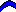icon of the Table toolbar. In order to create this type of graph you must select at least two Y columns of values (or a range from at least two columns). If there is an X column to the left of the Y columns, the X column values are used; otherwise, the worksheet's default X values are used. The area between the data plots (the two Y columns) is filled using a default blue fill pattern.

## Special Line/Symbol ->

### Vectors XYXY

Creates a vectors plot using the selected columns in the active table window. You must select four columns for this particular type of plot. The first two columns give the coordinates of the starting points of the vectors, the last two columns give the coordinates of the end points.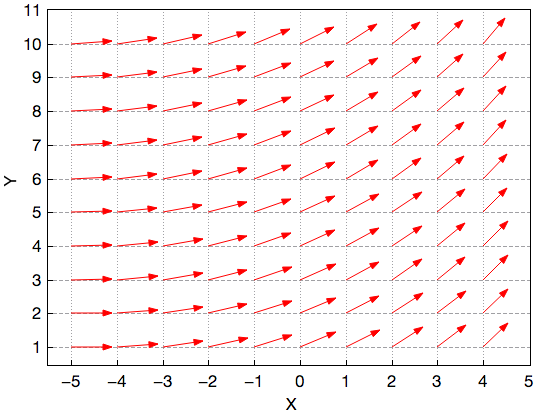### Vectors XYAM

Creates a vectors plot using the selected columns in the active table window. You must select four columns for this particular type of plot. The first two columns give the coordinates of the starting points of the vectors, the last two columns give the angles (in radians) and magnitudes of the vectors. It is also possible to define constant angle and magnitude values like in the example bellow showing an uniform vector field.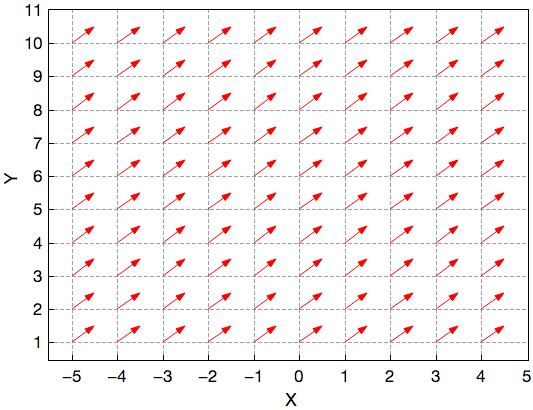### Zoom

Creates a new graph with 2 layers. The first is a standard plot layer which contains a curve for each selected Y column. The second is a special layer which has 2 components: 1) There is a standard plot layer that shows a portion of the first layer's surface in a magnified view; 2) There is a separate graphical window that can be sized and dragged around the first layer to specify the portion of that layer which is shown in the magnified view. The figure below shows a typical zoom plot.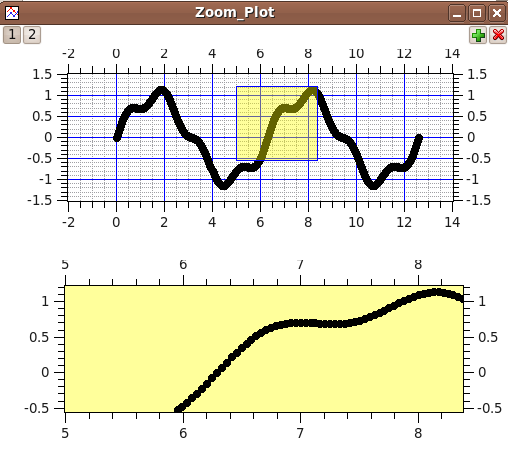## Statistical Graphs ->

Selecting the Statistical Graphs -> item opens up a sub-menu of commands for plotting various statistical graphs. Statistical plots do not give a direct drawing of the selected table data. However, a representation of the frequency distribution of the Y-values is plotted.

### Box Plot

Creates a box plot using the selected data columns in the active table window. This type of plot is used to give a graphical representation of some of the classical parameters of a frequency distribution, such as the mean, the min and max values, the position of the 95 and 5 percentiles, etc. The choice of the statistical parameters and the graphical parameters can be modified with the Custom curves dialog.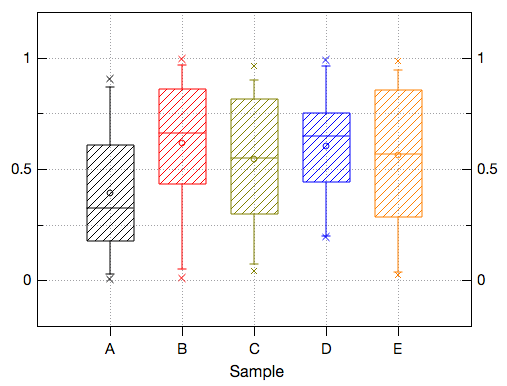### Box Plot + Data

Creates a box and data overlapped plot using the selected data columns in the active table window. This type of plot is used to give a graphical representation of some of the classical parameters of a frequency distribution, such as the mean, the min and max values, the position of the 95 and 5 percentiles, etc. The choice of the statistical parameters and the graphical parameters can be modified with the Custom curves dialog.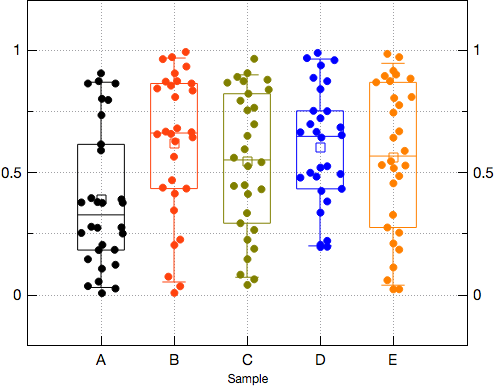### Interval Plot

Creates an interval plot using the selected data columns in the active table window. The interval plot is a box chart whose box type is set to Box, the box style is set to No Box and only displays the percentile symbol for the mean, drawn using a solid ellipse style. The range of the whiskers is set by default to Confidence Interval with a probability of 95% around the mean.### Scatter Interval

Creates a scatter interval plot using the selected data columns in the active table window. The scatter interval plot is a box chart whose box type is set to Box + Data, the box style is set to No Box. The range of the whiskers is set by default to SE (Standard Error) with a coefficient of 1.0 and the display of the mean line is enabled.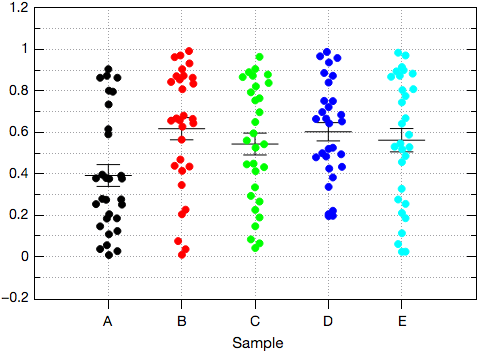### Violin Plot

Creates a violin plot using the selected data columns in the active table window. The violin plot is a special type of box chart, whose type is set to Box, the box style is set to No Box, the range of the whiskers is set to None and has a symmetric Kernel Smooth distribution curve overlayed on the binned data. The symmetric distribution curve has a semi transparent color filling.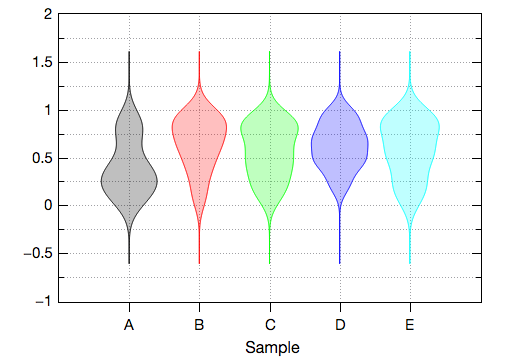### Violin with Box

Creates a violin with box plot using the selected data columns in the active table window. The violin with box plot is a box chart whose box type is set to Box, the box style is set to Rectangle, the range of the whiskers is set to MinMax and has a symmetric Kernel Smooth distribution curve overlayed on the binned data. The symmetric distribution curve has a semi transparent color filling.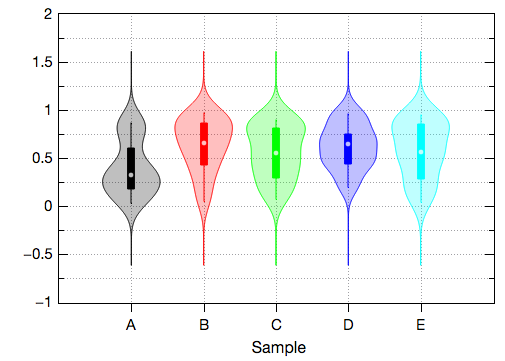### Ridgeline

Ridgeline curves are a special type of half violin plots, with exchanged X and Y axes. They can be quite useful when visualizing the distribution of several numeric variables. The partially overlapping distribution curves create the impression of a mountain range, which explains the name of this type of statistical plot. Their aspect and other properties can be customized using the Plot details... dialog.### Histogram

Creates a frequency histogram of the selected data columns in the active table window. The default binning uses 10 steps between the maximum and the minimum values. In order to customize the histogram double-click on the curve or right-click and select "Properties...". Both actions open the Plot Details dialog. The histogram controls are available on tabs on the right side of the dialog box. Binning behavior as well as the display of a distribution curve on the binned data can be customized via the Histogram Data tab.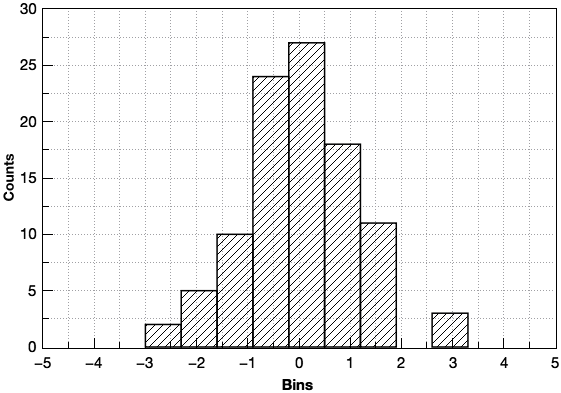Note that this command plots a frequency distribution that is computed from your data. If you want to draw a histogram directly from data values, use the Bars plot instead.

### Histogram + Probabilities

This command can be activated by clicking on the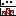icon of the Table toolbar. You must select exactly one Y column or a range from a table column. The Histogram + Probabilities creates a frequency histogram of the selected data column in the active table window, exactly like the Histogram command. Additionally, it plots a cumulative sum of observations in a second graph layer (layer 2). The statistical results - the mean, the standard deviation, the maximum and minimum values, and the total number of values - are also displayed to the Results log.

The two layers in the Histogram + Probabilities graph are linked. Therefore, before you make changes to the X axis scale or to the dimensions of the layer, make sure that the layer 2 is the active layer. This will ensure that the axis scales and dimensions of the layer 1 are adjusted accordingly.

### Histogram + Probabilities (Double-Y)

This command can be activated by clicking on theicon of the Table toolbar. You must select exactly one Y column or a range from a table column. The Histogram + Probabilities (Double-Y) creates a frequency histogram of the selected data column in the active table window, exactly like the Histogram command. Additionally, it plots a cumulative sum of observations using a vertical steps style. The cumulative percent curve is linked to the right Y axis of the graph layer, while the histogram is linked to the left Y axis.The statistical results - the mean, the standard deviation, the maximum and minimum values, and the total number of values - are also displayed to the Results log.

### Stacked Histogram

Creates vertically stacked layers displaying the histograms of the selected data columns in the active table window (one histogram per layer) See the Vertical 2 Layers command for more details.### Pareto Chart - Binned Data

This command can be activated by clicking on theicon of the Table toolbar. It creates a Pareto chart of the selected data column in the active table window. The input data typically consists of a text column containing the categories/items and one of numbers containing the corresponding counts or bins. You must select one or two columns.### Pareto Chart - Raw Data

This command can be activated by clicking on the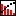icon of the Table toolbar. It creates a Pareto chart of the selected data column in the active table window. The input data typically consists of a text column containing the categories/items. QtiPlot counts the number of times each category/item occurs in the input data column and orders the results in decreasing order. You must select exactly one column.

### Q-Q Plot...

This command can be activated by clicking on theicon of the Table toolbar. It opens the Q-Q Plot dialog that allows to create and customize a Q-Q plot of the selected data column in the active table window. You must select a single column.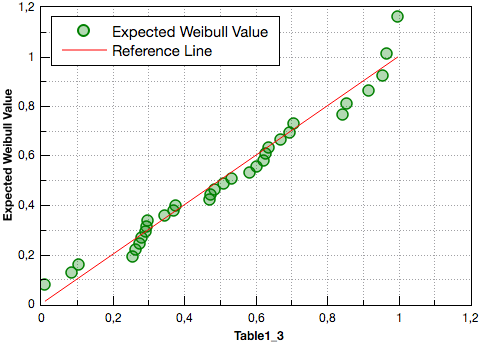### Stem and Leaf

Generates a stem-and-leaf tabular plot from the selected column in a new note window. Stem-and-Leaf plots are similar to histograms but are represented using rows of decimal digits. Like a histogram, data is binned, usually by taking the most significant digits (MSDs) as "stem" values which are listed in a column on the left. The least significant digit(s) from all values having identical MSDs are then listed to the right of each stem value. From a statistical point of view, this gives the appearance of a histogram but preserves the actual data values in the plot. When this command is executed, you will be given the opportunity to select/confirm the binning increment, which is given as a power of 10 and defaults to a power of 1 (that is, a binning increment of 10).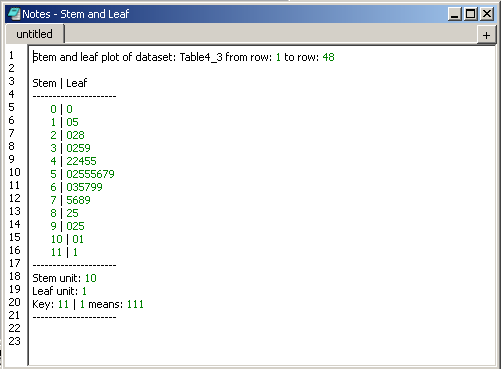This figure shows a typical stem-and-leaf representation of 48 random data points which are roughly Gaussian (i.e., normally distributed) and uses the default binning increment of 10.

## Panel ->

Selecting the Plot -> Panel -> menu item opens a sub-menu of commands that can be used to quickly obtain some commonly used arrangements of multiple plots. Unlike other commands in this menu, the new graph window that is created may have more than one layer (as needed).

### Vertical 2 Layers

Creates 2 vertically stacked layers. The data columns selected in the active table window (one curve per layer) are plotted on these layers.

### Horizontal 2 Layers

Creates 2 horizontally stacked layers. The data columns selected in the active table window (one curve per layer) are plotted on these layers.

### 4 Layers

Creates 4 layers on a 2x2 grid. The data columns selected in the active table window (one curve per layer) are plotted on these layers.

### Stacked Layers

Creates a group of vertically stacked layers, one layer per Y-Coordinate column selected. Data columns selected in the active table window (one curve per layer) are plotted on these layers.

### Custom Layout...

Opens the Arrange Layers dialog which allows complete customization of the layer layout.

## 3D XYY ->

Selecting the Plot -> 3D XYY -> menu item opens a sub-menu of commands used to draw some common 3D plots of XYY data columns from table windows. If the column selection do not include a X type column, the values for the X axis are automatically calculated by QtiPlot using row indices.

### Bars

Makes a 3D plot of the selected data columns in the active table window using Bars style.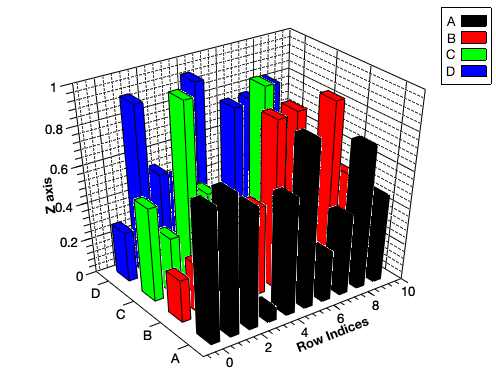### Inline Bars

Makes a 3D plot of the selected data columns in the active table window using Inline Bars style.### Stack Bars

Makes a 3D plot of the selected data columns in the active table window using Stack Bars style.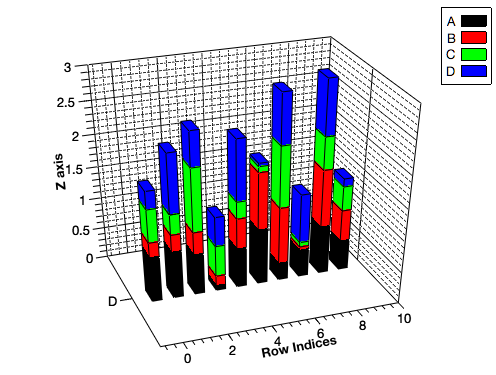### 100% Stack Bars

Makes a 3D plot of the selected data columns in the active table window using 100% Stack Bars style.### Ribbons

Makes a 3D plot of the selected data columns in the active table window using Ribbon style.### Wall

Makes a 3D plot of the selected data columns in the active table window using Wall style.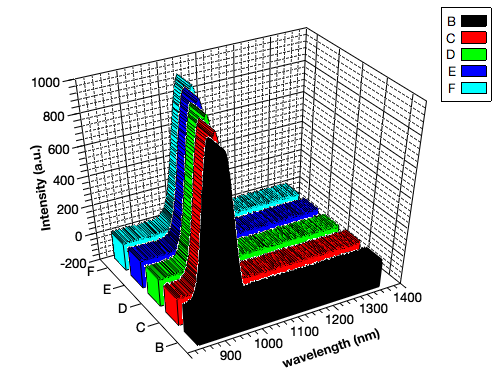### Stacked Wall

Makes a 3D plot of the selected data columns in the active table window using Stack Wall style.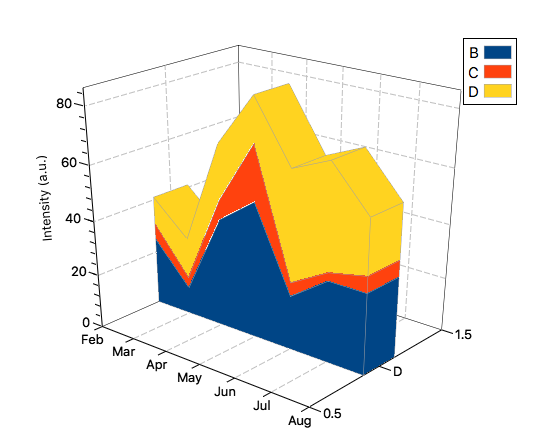### 100% Stacked Wall

Makes a 3D plot of the selected data columns in the active table window using 100% Stack Wall style.### Waterfall Plot

Makes a 3D plot of the selected data columns in the active table window using Waterfall style. The line color of each curve is determined by its index in the default Indexed colors list that can be customized via the Curves tab of the 2D Plots preferences dialog.

The default color used to fill the area bellow each curve, as well as the drawing of the side lines and of a base line can be customized using the controls in the Fill area under curve group box from the Curves tab of the 3D Plots preferences dialog.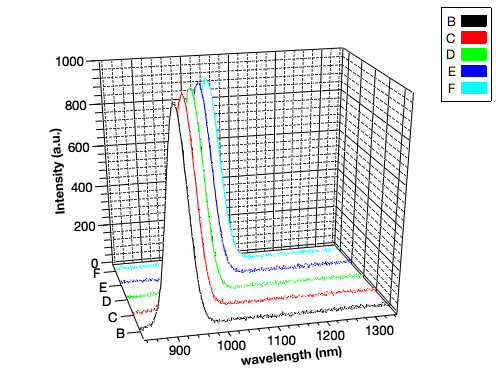### Waterfall Plot (Color Mapped)

Makes a 3D plot of the selected data columns in the active table window using Waterfall style. The line color of each curve is given by the default color map that can be customized via the Colors tab of the 3D Plots preferences dialog.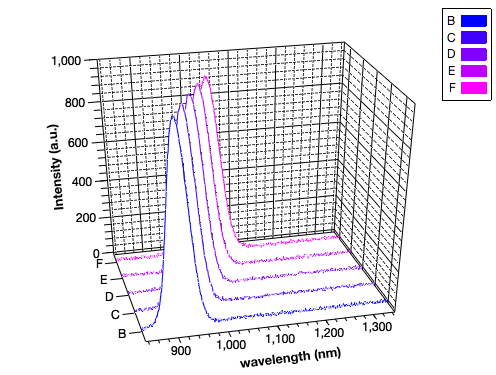The default color used to fill the area bellow each curve, as well as the drawing of the side lines and of a base line can be customized using the controls in the Fill area under curve group box from the Curves tab of the 3D Plots preferences dialog.

### Area

Makes a 3D plot of the selected data columns in the active table window using Area style. The default color used to fill the area bellow each curve is determined by the index of the curve in the default Indexed colors list that can be customized via the Curves tab of the 2D Plots preferences dialog.### Stack Area

Makes a 3D plot of the selected data columns in the active table window using Stack Area style.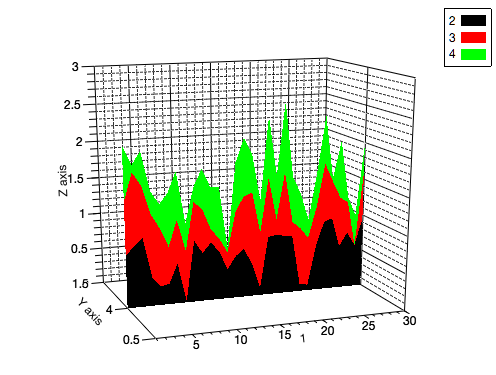### 100% Stack Area

Makes a 3D plot of the selected data columns in the active table window using 100% Stack Area style.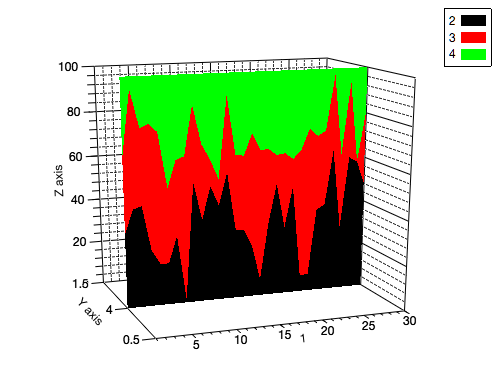### Trajectory

Makes a 3D plot of the selected data columns in the active table window using Trajectory style. The line color of each curve is determined by its index in the default Indexed colors list that can be customized via the Curves tab of the 2D Plots preferences dialog.The default line style and line width of the curves can be customized using the controls in the Mesh group box from the Curves tab of the 3D Plots preferences dialog.

### Scatter

Makes a 3D plot of the selected data columns in the active table window using Scatter style.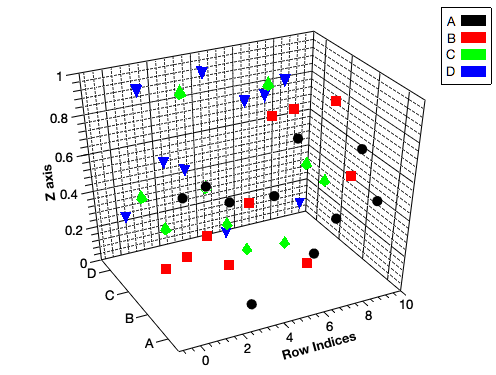### Trajectory + Dots

Makes a 3D plot of the selected data columns in the active table window using LineSymbols style. The line color of each curve is determined by its index in the default Indexed colors list that can be customized via the Curves tab of the 2D Plots preferences dialog.The default line style and line width of the curves can be customized using the controls in the Mesh group box from the Curves tab of the 3D Plots preferences dialog.

## 3D Plot ->

Selecting the Plot -> 3D Plot -> menu item opens a sub-menu of commands used to draw some common 3D plots of 2D data.

### Ribbons

Makes a 3D plot of the selected data column in the active table window (only one column allowed) using Ribbon style.### Bars

Makes a 3D plot of the selected data column in the active table window (only one column allowed) using "3D Bars" style.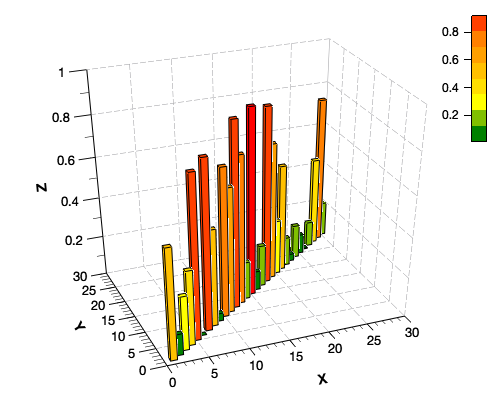### Scatter

Makes a 3D plot of the selected data column in the active table window (only one column allowed) using "3D Dots" style. The 3D point symbol style can be changed via the 3D Plots Settings dialog.With scatter plots, you can choose the kind of graphic item which is used to plot the data points. The example above is done with dots, but you can also select cross hairs or cones. This can be done either with the corresponding icons of the 3D plot toolbar (respectivelyand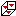for cross-hairs, dots and cones) or with the Data tab of the 3D plot options dialog.

### Trajectory

Makes a 3D plot of the selected data column in the active table window (only one column allowed) using "3D Line" style. The line width and color may be changed via the 3D Plot Settings dialog.### Trajectory + Dots

Makes a 3D plot of the selected data column in the active table window (only one column allowed) using a line and dot symbols style. The default properties of the line (width and color) and of the symbols (style, size, etc...) may be changed via the 3D Plots Settings dialog.### Vectors XYZ XYZ

Makes a 3D vectors plot of the selected data columns in the active table window (six columns needed). The XYZ XYZ data in the six columns define the start and end points for the vectors. The line width, color and other properties of the vectors may be changed via the 3D Vectors tab of the 3D Plot Settings dialog.

### Vectors XYZ dXdYdZ

Makes a 3D vectors plot of the selected data columns in the active table window (six columns needed). The XYZ dXdYdZ data columns provide the vector start points and the distances between the projections of the start and end points of the vectors on the three axes, X, Y and Z, respectively. The line width, color and other properties of the vectors may be changed via the 3D Vectors tab of the 3D Plot Settings dialog.